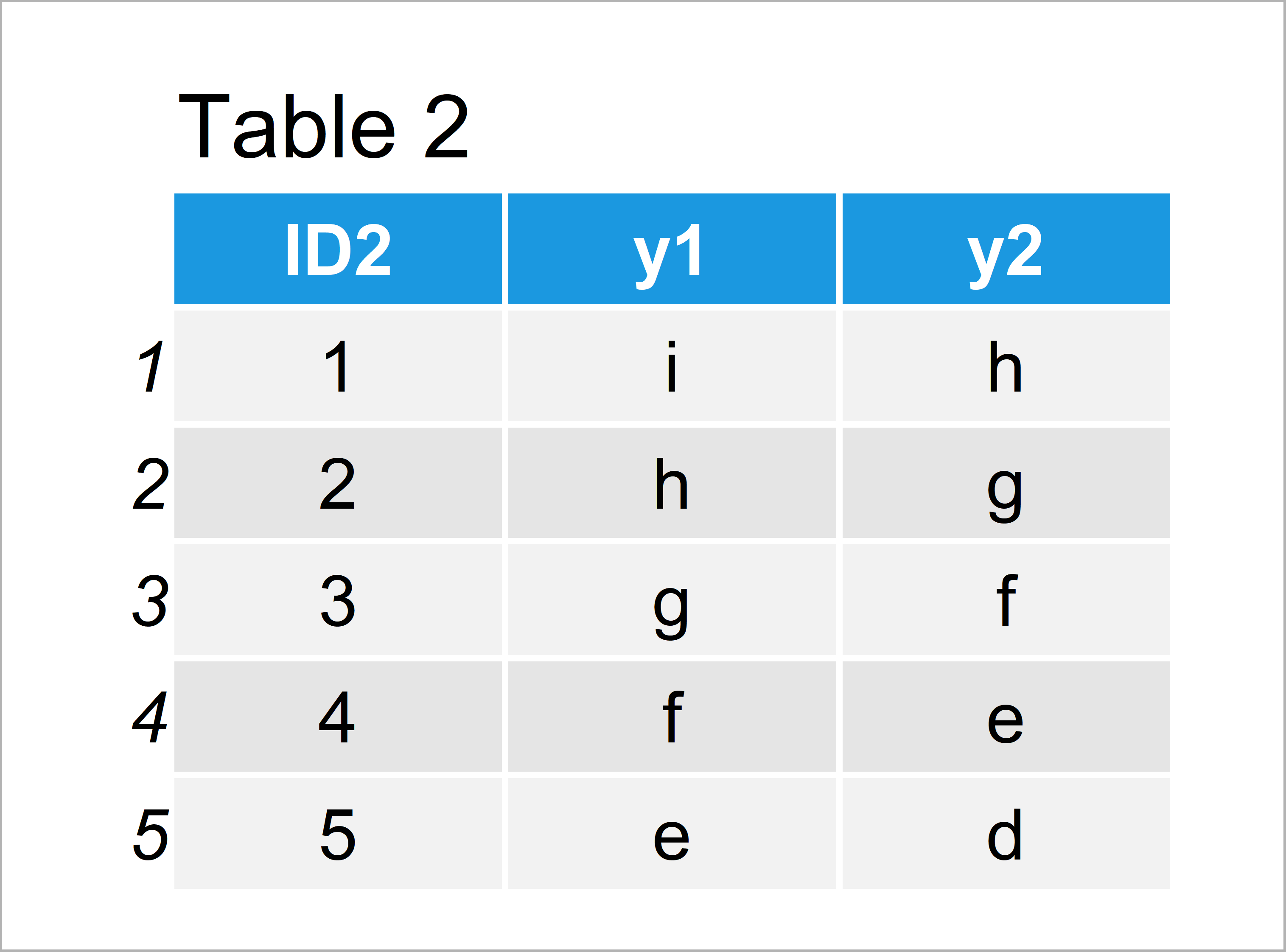# R merge Error in fix.by(by.y, y) : ‘by’ must specify a uniquely valid column

In this tutorial you’ll learn how to handle the “Error in fix.by(by.y, y) : ‘by’ must specify a uniquely valid column” in the R programming language.

The tutorial will consist of the following content:

Let’s dive right into the R syntax!

## Creating Example Data

Initially, we’ll have to create two data frames that we can use in the examples below. Our first example data frame looks as follows:

```data1 <- data.frame(ID1 = 1:5, # Create example data 1 x1 = 9:5, x2 = 8:4) data1 # Print example data 1```Have a look at the table that has been returned by the previous R syntax. It shows that our first example data frame consists of five rows and three columns.

Let’s create another data frame in R:

```data2 <- data.frame(ID2 = 1:5, # Create example data 2 y1 = letters[9:5], y2 = letters[8:4]) data2 # Print example data 2```As shown in Table 2, we have created a second data frame by running the previous R programming code.

Our second data frame also consists of five rows and three columns. However, the variable names are different.

Let’s combine these data!

## Example 1: Reproduce the Error in fix.by(by.y, y) : ‘by’ must specify a uniquely valid column

The R programming syntax below shows how to replicate the error message “Error in fix.by(by.y, y) : ‘by’ must specify a uniquely valid column” when using the merge function in R.

Consider the following R code:

```data_all <- merge(data1, # Try to merge data data2, by = "ID1") # Error in fix.by(by.y, y) : 'by' must specify a uniquely valid column```

As you can see, the previous R code has returned the error message “Error in fix.by(by.y, y) : ‘by’ must specify a uniquely valid column”.

The reason for this is that the ID variable is named differently in both data frames. However, we have not specified that properly within the merge function.

Next, I’ll show how to solve this problem. So keep on reading!

## Example 2: Fix the Error in fix.by(by.y, y) : ‘by’ must specify a uniquely valid column

The following code explains how to properly specify different ID columns when merging data frames to avoid the “Error in fix.by(by.y, y) : ‘by’ must specify a uniquely valid column”.

Have a look at the following specification of the two different ID columns:

```data_all <- merge(data1, # Properly merge data data2, by.x = "ID1", by.y = "ID2") data_all # Print merged data```As shown in Table 3, the previous R programming syntax has created a joined data frame containing the values of both input data frames.

## Video, Further Resources & Summary

Do you need further explanations on the R code of the present article? Then I recommend watching the following video of my YouTube channel. In the video instruction, I’m explaining the R programming code of this article.

Furthermore, you may have a look at the other tutorials of my website. I have published numerous tutorials already:

Summary: In this R programming tutorial you have learned how to deal with the “Error in fix.by(by.y, y) : ‘by’ must specify a uniquely valid column”. Let me know in the comments, if you have further questions.

Subscribe to the Statistics Globe Newsletter